Elementary Algebra

Elementary Algebra1.3 Add and Subtract Integers

### Learning Objectives

By the end of this section, you will be able to:

• Use negatives and opposites
• Simplify: expressions with absolute value
• Subtract integers

### Be Prepared 1.3

A more thorough introduction to the topics covered in this section can be found in the Prealgebra chapter, Integers.

### Use Negatives and Opposites

Our work so far has only included the counting numbers and the whole numbers. But if you have ever experienced a temperature below zero or accidentally overdrawn your checking account, you are already familiar with negative numbers. Negative numbers are numbers less than $0.0.$ The negative numbers are to the left of zero on the number line. See Figure 1.6.

Figure 1.6 The number line shows the location of positive and negative numbers.

The arrows on the ends of the number line indicate that the numbers keep going forever. There is no biggest positive number, and there is no smallest negative number.

Is zero a positive or a negative number? Numbers larger than zero are positive, and numbers smaller than zero are negative. Zero is neither positive nor negative.

Consider how numbers are ordered on the number line. Going from left to right, the numbers increase in value. Going from right to left, the numbers decrease in value. See Figure 1.7.

Figure 1.7 The numbers on a number line increase in value going from left to right and decrease in value going from right to left.

### Manipulative Mathematics

Doing the Manipulative Mathematics activity “Number Line-part 2” will help you develop a better understanding of integers.

Remember that we use the notation:

a < b (read “a is less than b”) when a is to the left of b on the number line.

a > b (read “a is greater than b”) when a is to the right of b on the number line.

Now we need to extend the number line which showed the whole numbers to include negative numbers, too. The numbers marked by points in Figure 1.8 are called the integers. The integers are the numbers $…−3,−2,−1,0,1,2,3……−3,−2,−1,0,1,2,3…$

Figure 1.8 All the marked numbers are called integers.

### Example 1.30

Order each of the following pairs of numbers, using < or >: $14___614___6$ $−1___9−1___9$ $−1___−4−1___−4$ $2___−20.2___−20.$

### Try It 1.59

Order each of the following pairs of numbers, using $<<$ or $>:>:$ $15___715___7$ $−2___5−2___5$ $−3___−7−3___−7$
$5___−17.5___−17.$

### Try It 1.60

Order each of the following pairs of numbers, using $<<$ or $>:>:$ $8___138___13$ $3___−43___−4$ $−5___−2−5___−2$
$9___−21.9___−21.$

You may have noticed that, on the number line, the negative numbers are a mirror image of the positive numbers, with zero in the middle. Because the numbers 2 and $−2−2$ are the same distance from zero, they are called opposites. The opposite of 2 is $−2,−2,$ and the opposite of $−2−2$ is 2.

### Opposite

The opposite of a number is the number that is the same distance from zero on the number line but on the opposite side of zero.

Figure 1.9 illustrates the definition.

Figure 1.9 The opposite of 3 is $−3.−3.$

Sometimes in algebra the same symbol has different meanings. Just like some words in English, the specific meaning becomes clear by looking at how it is used. You have seen the symbol “−” used in three different ways.

$10−4Between two numbers, it indicates the operation ofsubtraction.We read10−4as“10minus4.”−8In front of a number, it indicates anegativenumber.We read−8as “negative eight.”−xIn front of a variable, it indicates theopposite.We read−xas “the opposite ofx.”−(−2)Here there are two“−”signs. The one in the parentheses tells us the number isnegative2.The one outside the parentheses tells us to take theoppositeof−2.We read−(−2)as “the opposite of negative two.”10−4Between two numbers, it indicates the operation ofsubtraction.We read10−4as“10minus4.”−8In front of a number, it indicates anegativenumber.We read−8as “negative eight.”−xIn front of a variable, it indicates theopposite.We read−xas “the opposite ofx.”−(−2)Here there are two“−”signs. The one in the parentheses tells us the number isnegative2.The one outside the parentheses tells us to take theoppositeof−2.We read−(−2)as “the opposite of negative two.”$
 $10−410−4$ Between two numbers, it indicates the operation of subtraction.We read $10−410−4$ as "10 minus 4." $−8−8$ In front of a number, it indicates a negative number. We read −8 as "negative eight." $−x−x$ In front of a variable, it indicates the opposite. We read $−x−x$ as "the opposite of $xx$." $−(−2)−(−2)$ Here there are two "−" signs. The one in the parentheses tells us the number is negative 2. The one outside the parentheses tells us to take the opposite of −2. We read $−(−2)−(−2)$ as "the opposite of negative two."
Table 1.6

### Opposite Notation

$−a−a$ means the opposite of the number a.

The notation $−a−a$ is read as “the opposite of a.”

### Example 1.31

Find: the opposite of 7 the opposite of $−10−10$ $−(−6).−(−6).$

### Try It 1.61

Find: the opposite of 4 the opposite of $−3−3$ $−(−1).−(−1).$

### Try It 1.62

Find: the opposite of 8 the opposite of $−5−5$ $−(−5).−(−5).$

Our work with opposites gives us a way to define the integers.The whole numbers and their opposites are called the integers. The integers are the numbers $…−3,−2,−1,0,1,2,3……−3,−2,−1,0,1,2,3…$

### Integers

The whole numbers and their opposites are called the integers.

The integers are the numbers

$…−3,−2,−1,0,1,2,3……−3,−2,−1,0,1,2,3…$

When evaluating the opposite of a variable, we must be very careful. Without knowing whether the variable represents a positive or negative number, we don’t know whether $−x−x$ is positive or negative. We can see this in Example 1.32.

### Example 1.32

Evaluate $−x,−x,$ when $x=8x=8$ $−x,−x,$ when $x=−8.x=−8.$

### Try It 1.63

Evaluate $−n,−n,$ when $n=4n=4$ $n=−4.n=−4.$

### Try It 1.64

Evaluate $−m,−m,$ when $m=11m=11$ $m=−11.m=−11.$

### Simplify: Expressions with Absolute Value

We saw that numbers such as $2and−22and−2$ are opposites because they are the same distance from 0 on the number line. They are both two units from 0. The distance between 0 and any number on the number line is called the absolute value of that number.

### Absolute Value

The absolute value of a number is its distance from 0 on the number line.

The absolute value of a number n is written as $|n|.|n|.$

For example,

• $−5is5−5is5$ units away from $0,0,$ so $|−5|=5.|−5|=5.$
• $5is55is5$ units away from $0,0,$ so $|5|=5.|5|=5.$

Figure 1.10 illustrates this idea.

Figure 1.10 The integers $5and are55and are5$ units away from $0.0.$

The absolute value of a number is never negative (because distance cannot be negative). The only number with absolute value equal to zero is the number zero itself, because the distance from $0to00to0$ on the number line is zero units.

### Property of Absolute Value

$|n|≥0|n|≥0$ for all numbers

Absolute values are always greater than or equal to zero!

Mathematicians say it more precisely, “absolute values are always non-negative.” Non-negative means greater than or equal to zero.

### Example 1.33

Simplify: $|3||3|$ $|−44||−44|$ $|0||0|$.

### Try It 1.65

Simplify: $|4||4|$ $|−28||−28|$ $|0|.|0|.$

### Try It 1.66

Simplify: $|−13||−13|$ $|47||47|$ $|0|.|0|.$

In the next example, we’ll order expressions with absolute values. Remember, positive numbers are always greater than negative numbers!

### Example 1.34

Fill in $<,>,or=<,>,or=$ for each of the following pairs of numbers:

$|−5|___−|−5||−5|___−|−5|$ $8___−|−8|8___−|−8|$ $−9___−|−9|−9___−|−9|$ $−(−16)___−|−16|−(−16)___−|−16|$

### Try It 1.67

Fill in <, >, or $==$ for each of the following pairs of numbers: $|−9|___−|−9||−9|___−|−9|$ $2___−|−2|2___−|−2|$ $−8___|−8|−8___|−8|$
$−(−9)___−|−9|.−(−9)___−|−9|.$

### Try It 1.68

Fill in <, >, or $==$ for each of the following pairs of numbers: $7___−|−7|7___−|−7|$ $−(−10)___−|−10|−(−10)___−|−10|$
$|−4|___−|−4||−4|___−|−4|$ $−1___|−1|.−1___|−1|.$

We now add absolute value bars to our list of grouping symbols. When we use the order of operations, first we simplify inside the absolute value bars as much as possible, then we take the absolute value of the resulting number.

### Grouping Symbols

$Parentheses()Braces{}Brackets[]Absolute value||Parentheses()Braces{}Brackets[]Absolute value||$

In the next example, we simplify the expressions inside absolute value bars first, just like we do with parentheses.

### Example 1.35

Simplify: $24−|19−3(6−2)|.24−|19−3(6−2)|.$

### Try It 1.69

Simplify: $19−|11−4(3−1)|.19−|11−4(3−1)|.$

### Try It 1.70

Simplify: $9−|8−4(7−5)|.9−|8−4(7−5)|.$

### Example 1.36

Evaluate: $|x|whenx=−35|x|whenx=−35$ $|−y|wheny=−20|−y|wheny=−20$ $−|u|whenu=12−|u|whenu=12$ $−|p|whenp=−14.−|p|whenp=−14.$

### Try It 1.71

Evaluate: $|x|whenx=−17|x|whenx=−17$ $|−y|wheny=−39|−y|wheny=−39$ $−|m|whenm=22−|m|whenm=22$ $−|p|whenp=−11.−|p|whenp=−11.$

### Try It 1.72

Evaluate: $|y|wheny=−23|y|wheny=−23$ $|−y|wheny=−21|−y|wheny=−21$ $−|n|whenn=37−|n|whenn=37$ $−|q|whenq=−49.−|q|whenq=−49.$

Most students are comfortable with the addition and subtraction facts for positive numbers. But doing addition or subtraction with both positive and negative numbers may be more challenging.

### Manipulative Mathematics

We will use two color counters to model addition and subtraction of negatives so that you can visualize the procedures instead of memorizing the rules.

We let one color (blue) represent positive. The other color (red) will represent the negatives. If we have one positive counter and one negative counter, the value of the pair is zero. They form a neutral pair. The value of this neutral pair is zero.We will use the counters to show how to add the four addition facts using the numbers $5,−55,−5$ and $3,−3.3,−3.$

$5+3−5+(−3)−5+35+(−3)5+3−5+(−3)−5+35+(−3)$

To add $5+3,5+3,$ we realize that $5+35+3$ means the sum of 5 and 3.

 We start with 5 positives.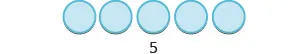And then we add 3 positives.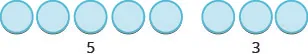We now have 8 positives. The sum of 5 and 3 is 8.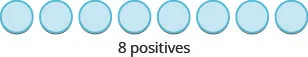Now we will add $−5+(−3).−5+(−3).$ Watch for similarities to the last example $5+3=8.5+3=8.$

To add $−5+(−3),−5+(−3),$ we realize this means the sum of $−5and−3.−5and−3.$

 We start with 5 negatives.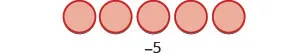And then we add 3 negatives.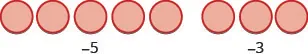We now have 8 negatives. The sum of −5 and −3 is −8.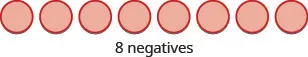In what ways were these first two examples similar?

• The first example adds 5 positives and 3 positives—both positives.
• The second example adds 5 negatives and 3 negatives—both negatives.

In each case we got 8—either 8 positives or 8 negatives.

When the signs were the same, the counters were all the same color, and so we added them.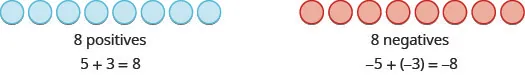### Example 1.37

Add: $1+41+4$ $−1+(−4).−1+(−4).$

### Try It 1.73

Add: $2+42+4$ $−2+(−4).−2+(−4).$

### Try It 1.74

Add: $2+52+5$ $−2+(−5).−2+(−5).$

So what happens when the signs are different? Let’s add $−5+3.−5+3.$ We realize this means the sum of $−5−5$ and 3. When the counters were the same color, we put them in a row. When the counters are a different color, we line them up under each other.

 −5 + 3 means the sum of −5 and 3. We start with 5 negatives.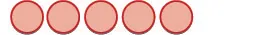And then we add 3 positives.We remove any neutral pairs.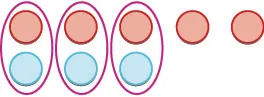We have 2 negatives left.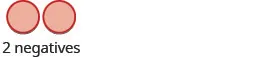The sum of −5 and 3 is −2. −5 + 3 = −2

Notice that there were more negatives than positives, so the result was negative.

Let’s now add the last combination, $5+(−3).5+(−3).$

 5 + (−3) means the sum of 5 and −3. We start with 5 positives.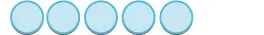And then we add 3 negatives.We remove any neutral pairs.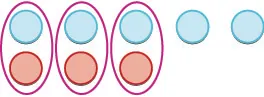We have 2 positives left.The sum of 5 and −3 is 2. 5 + (−3) = 2

When we use counters to model addition of positive and negative integers, it is easy to see whether there are more positive or more negative counters. So we know whether the sum will be positive or negative.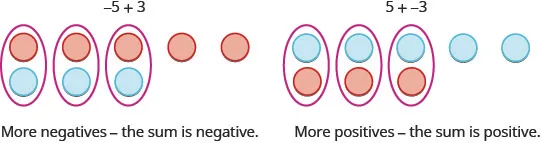### Example 1.38

Add: $−1+5−1+5$ $1+(−5).1+(−5).$

### Try It 1.75

Add: $−2+4−2+4$ $2+(−4).2+(−4).$

### Try It 1.76

Add: $−2+5−2+5$ $2+(−5).2+(−5).$

Now that we have added small positive and negative integers with a model, we can visualize the model in our minds to simplify problems with any numbers.

When you need to add numbers such as $37+(−53),37+(−53),$ you really don’t want to have to count out 37 blue counters and 53 red counters. With the model in your mind, can you visualize what you would do to solve the problem?

Picture 37 blue counters with 53 red counters lined up underneath. Since there would be more red (negative) counters than blue (positive) counters, the sum would be negative. How many more red counters would there be? Because $53−37=16,53−37=16,$ there are 16 more red counters.

Therefore, the sum of $37+(−53)37+(−53)$ is $−16.−16.$

$37+(−53)=−1637+(−53)=−16$

Let’s try another one. We’ll add $−74+(−27).−74+(−27).$ Again, imagine 74 red counters and 27 more red counters, so we’d have 101 red counters. This means the sum is $−101.−101.$

$−74+(−27)=−101−74+(−27)=−101$

Let’s look again at the results of adding the different combinations of $5,−55,−5$ and $3,−3.3,−3.$

### Addition of Positive and Negative Integers

$5+3−5+(−3)8−8both positive, sum positiveboth negative, sum negative5+3−5+(−3)8−8both positive, sum positiveboth negative, sum negative$

When the signs are the same, the counters would be all the same color, so add them.

$−5+35+(−3)−22different signs, more negatives, sum negativedifferent signs, more positives, sum positive−5+35+(−3)−22different signs, more negatives, sum negativedifferent signs, more positives, sum positive$

When the signs are different, some of the counters would make neutral pairs, so subtract to see how many are left.

Visualize the model as you simplify the expressions in the following examples.

### Example 1.39

Simplify: $19+(−47)19+(−47)$ $−14+(−36).−14+(−36).$

### Try It 1.77

Simplify: $−31+(−19)−31+(−19)$ $15+(−32).15+(−32).$

### Try It 1.78

Simplify: $−42+(−28)−42+(−28)$ $25+(−61).25+(−61).$

The techniques used up to now extend to more complicated problems, like the ones we’ve seen before. Remember to follow the order of operations!

### Example 1.40

Simplify: $−5+3(−2+7).−5+3(−2+7).$

### Try It 1.79

Simplify: $−2+5(−4+7).−2+5(−4+7).$

### Try It 1.80

Simplify: $−4+2(−3+5).−4+2(−3+5).$

### Manipulative Mathematics

Doing the Manipulative Mathematics activity “Subtraction of Signed Numbers” will help you develop a better understanding of subtracting integers.

We will continue to use counters to model the subtraction. Remember, the blue counters represent positive numbers and the red counters represent negative numbers.

Perhaps when you were younger, you read $“5−3”“5−3”$ as $“5“5$ take away $3.”3.”$ When you use counters, you can think of subtraction the same way!

We will model the four subtraction facts using the numbers $55$ and $3.3.$

$5−3−5−(−3)−5−35−(−3)5−3−5−(−3)−5−35−(−3)$

To subtract $5−3,5−3,$ we restate the problem as $“5“5$ take away $3.”3.”$

 We start with 5 positives.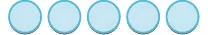We ‘take away’ 3 positives.We have 2 positives left. The difference of 5 and 3 is 2. 2

Now we will subtract $−5−(−3).−5−(−3).$ Watch for similarities to the last example $5−3=2.5−3=2.$

To subtract $−5−(−3),−5−(−3),$ we restate this as $“–5“–5$ take away $–3”–3”$

 We start with 5 negatives.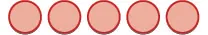We ‘take away’ 3 negatives.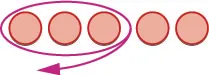We have 2 negatives left. The difference of −5 and −3 is −2. −2

Notice that these two examples are much alike: The first example, we subtract 3 positives from 5 positives and end up with 2 positives.

In the second example, we subtract 3 negatives from 5 negatives and end up with 2 negatives.

Each example used counters of only one color, and the “take away” model of subtraction was easy to apply.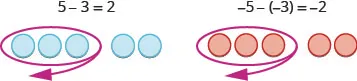### Example 1.41

Subtract: $7−57−5$ $−7−(−5).−7−(−5).$

### Try It 1.81

Subtract: $6−46−4$ $−6−(−4).−6−(−4).$

### Try It 1.82

Subtract: $7−47−4$ $−7−(−4).−7−(−4).$

What happens when we have to subtract one positive and one negative number? We’ll need to use both white and red counters as well as some neutral pairs. Adding a neutral pair does not change the value. It is like changing quarters to nickels—the value is the same, but it looks different.

• To subtract $−5−3,−5−3,$ we restate it as $−5−5$ take away 3.

We start with 5 negatives. We need to take away 3 positives, but we do not have any positives to take away.

Remember, a neutral pair has value zero. If we add 0 to 5 its value is still 5. We add neutral pairs to the 5 negatives until we get 3 positives to take away.

 −5 − 3 means −5 take away 3. We start with 5 negatives.We now add the neutrals needed to get 3 positives.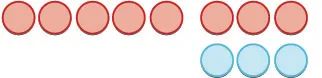We remove the 3 positives.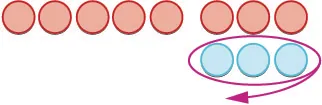We are left with 8 negatives.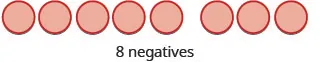The difference of −5 and 3 is −8. −5 − 3 = −8

And now, the fourth case, $5−(−3).5−(−3).$ We start with 5 positives. We need to take away 3 negatives, but there are no negatives to take away. So we add neutral pairs until we have 3 negatives to take away.

 5 − (−3) means 5 take away −3. We start with 5 positives.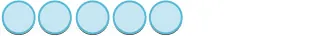We now add the needed neutrals pairs.We remove the 3 negatives.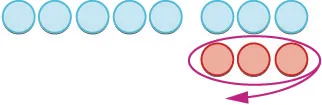We are left with 8 positives.The difference of 5 and −3 is 8. 5 − (−3) = 8

### Example 1.42

Subtract: $−3−1−3−1$ $3−(−1).3−(−1).$

### Try It 1.83

Subtract: $−6−4−6−4$ $6−(−4).6−(−4).$

### Try It 1.84

Subtract: $−7−4−7−4$ $7−(−4).7−(−4).$

Have you noticed that subtraction of signed numbers can be done by adding the opposite? In Example 1.42, $−3−1−3−1$ is the same as $−3+(−1)−3+(−1)$ and $3−(−1)3−(−1)$ is the same as $3+1.3+1.$ You will often see this idea, the subtraction property, written as follows:

### Subtraction Property

$a−b=a+(−b)a−b=a+(−b)$

Subtracting a number is the same as adding its opposite.

Look at these two examples.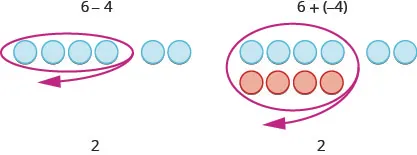$6−4gives the same answer as6+(−4).6−4gives the same answer as6+(−4).$

Of course, when you have a subtraction problem that has only positive numbers, like $6−4,6−4,$ you just do the subtraction. You already knew how to subtract $6−46−4$ long ago. But knowing that $6−46−4$ gives the same answer as $6+(−4)6+(−4)$ helps when you are subtracting negative numbers. Make sure that you understand how $6−46−4$ and $6+(−4)6+(−4)$ give the same results!

### Example 1.43

Simplify: $13−813−8$ and $13+(−8)13+(−8)$ $−17−9−17−9$ and $−17+(−9).−17+(−9).$

### Try It 1.85

Simplify: $21−1321−13$ and $21+(−13)21+(−13)$ $−11−7−11−7$ and $−11+(−7).−11+(−7).$

### Try It 1.86

Simplify: $15−715−7$ and $15+(−7)15+(−7)$ $−14−8−14−8$ and $−14+(−8).−14+(−8).$

Look at what happens when we subtract a negative.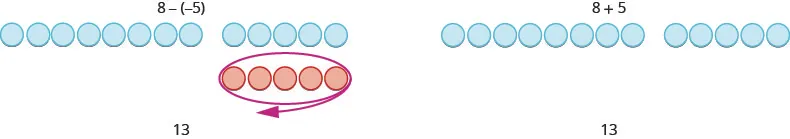$8−(−5)gives the same answer as8+58−(−5)gives the same answer as8+5$

Subtracting a negative number is like adding a positive!

You will often see this written as $a−(−b)=a+b.a−(−b)=a+b.$

Does that work for other numbers, too? Let’s do the following example and see.

### Example 1.44

Simplify: $9−(−15)9−(−15)$ and $9+159+15$ $−7−(−4)−7−(−4)$ and $−7+4.−7+4.$

### Try It 1.87

Simplify: $6−(−13)6−(−13)$ and $6+136+13$ $−5−(−1)−5−(−1)$ and $−5+1.−5+1.$

### Try It 1.88

Simplify: $4−(−19)4−(−19)$ and $4+194+19$ $−4−(−7)−4−(−7)$ and $−4+7.−4+7.$

Let’s look again at the results of subtracting the different combinations of $5,−55,−5$ and $3,−3.3,−3.$

### Subtraction of Integers

$5−3−5−(−3)2−25positives take away3positives5negatives take away3negatives2positives2negatives5−3−5−(−3)2−25positives take away3positives5negatives take away3negatives2positives2negatives$

When there would be enough counters of the color to take away, subtract.

$−5−35−(−3)−885negatives, want to take away3positives5positives, want to take away3negativesneed neutral pairsneed neutral pairs−5−35−(−3)−885negatives, want to take away3positives5positives, want to take away3negativesneed neutral pairsneed neutral pairs$

When there would be not enough counters of the color to take away, add.

What happens when there are more than three integers? We just use the order of operations as usual.

### Example 1.45

Simplify: $7−(−4−3)−9.7−(−4−3)−9.$

### Try It 1.89

Simplify: $8−(−3−1)−98−(−3−1)−9$.

### Try It 1.90

Simplify: $12−(−9−6)−1412−(−9−6)−14$.

### Section 1.3 Exercises

#### Practice Makes Perfect

Use Negatives and Opposites of Integers

In the following exercises, order each of the following pairs of numbers, using < or >.

185.

$9___49___4$
$−3___6−3___6$
$−8___−2−8___−2$
$1___−101___−10$

186.

$−7___3−7___3$
$−10___−5−10___−5$
$2___−62___−6$
$8___98___9$

In the following exercises, find the opposite of each number.

187.

2
$−6−6$

188.

9
$−4−4$

In the following exercises, simplify.

189.

$− ( −4 ) − ( −4 )$

190.

$− ( −8 ) − ( −8 )$

191.

$− ( −15 ) − ( −15 )$

192.

$− ( −11 ) − ( −11 )$

In the following exercises, evaluate.

193.

$−c−c$ when
$c=12c=12$
$c=−12c=−12$

194.

$−d−d$ when
$d=21d=21$
$d=−21d=−21$

Simplify Expressions with Absolute Value

In the following exercises, simplify.

195.

$|−32||−32|$
$|0||0|$
$|16||16|$

196.

$|0||0|$
$|−40||−40|$
$|22||22|$

In the following exercises, fill in <, >, or $==$ for each of the following pairs of numbers.

197.

$−6___|−6|−6___|−6|$
$−|−3|___−3−|−3|___−3$

198.

$|−5|___−|−5||−5|___−|−5|$
$9___−|−9|9___−|−9|$

In the following exercises, simplify.

199.

$− ( −5 ) and − | −5 | − ( −5 ) and − | −5 |$

200.

$− | −9 | and − ( −9 ) − | −9 | and − ( −9 )$

201.

$8 | −7 | 8 | −7 |$

202.

$5 | −5 | 5 | −5 |$

203.

$| 15 − 7 | − | 14 − 6 | | 15 − 7 | − | 14 − 6 |$

204.

$| 17 − 8 | − | 13 − 4 | | 17 − 8 | − | 13 − 4 |$

205.

$18 − | 2 ( 8 − 3 ) | 18 − | 2 ( 8 − 3 ) |$

206.

$18 − | 3 ( 8 − 5 ) | 18 − | 3 ( 8 − 5 ) |$

In the following exercises, evaluate.

207.

$−|p|whenp=19−|p|whenp=19$
$−|q|whenq=−33−|q|whenq=−33$

208.

$−|a|whena=60−|a|whena=60$
$−|b|whenb=−12−|b|whenb=−12$

In the following exercises, simplify each expression.

209.

$−21 + ( −59 ) −21 + ( −59 )$

210.

$−35 + ( −47 ) −35 + ( −47 )$

211.

$48 + ( −16 ) 48 + ( −16 )$

212.

$34 + ( −19 ) 34 + ( −19 )$

213.

$−14 + ( −12 ) + 4 −14 + ( −12 ) + 4$

214.

$−17 + ( −18 ) + 6 −17 + ( −18 ) + 6$

215.

$135 + ( −110 ) + 83 135 + ( −110 ) + 83$

216.

$−38 + 27 + ( −8 ) + 12 −38 + 27 + ( −8 ) + 12$

217.

$19 + 2 ( −3 + 8 ) 19 + 2 ( −3 + 8 )$

218.

$24 + 3 ( −5 + 9 ) 24 + 3 ( −5 + 9 )$

Subtract Integers

In the following exercises, simplify.

219.

$8 − 2 8 − 2$

220.

$−6 − ( −4 ) −6 − ( −4 )$

221.

$−5 − 4 −5 − 4$

222.

$−7 − 2 −7 − 2$

223.

$8 − ( −4 ) 8 − ( −4 )$

224.

$7 − ( −3 ) 7 − ( −3 )$

225.

$44−2844−28$
$44+(−28)44+(−28)$

226.

$35−1635−16$
$35+(−16)35+(−16)$

227.

$27−(−18)27−(−18)$
$27+1827+18$

228.

$46−(−37)46−(−37)$
$46+3746+37$

In the following exercises, simplify each expression.

229.

$15 − ( −12 ) 15 − ( −12 )$

230.

$14 − ( −11 ) 14 − ( −11 )$

231.

$48 − 87 48 − 87$

232.

$45 − 69 45 − 69$

233.

$−17 − 42 −17 − 42$

234.

$−19 − 46 −19 − 46$

235.

$−103 − ( −52 ) −103 − ( −52 )$

236.

$−105 − ( −68 ) −105 − ( −68 )$

237.

$−45 − ( –54 ) −45 − ( –54 )$

238.

$−58 − ( −67 ) −58 − ( −67 )$

239.

$8 − 3 − 7 8 − 3 − 7$

240.

$9 − 6 − 5 9 − 6 − 5$

241.

$−5 − 4 + 7 −5 − 4 + 7$

242.

$−3 − 8 + 4 −3 − 8 + 4$

243.

$−14 − ( −27 ) + 9 −14 − ( −27 ) + 9$

244.

$64 + ( −17 ) − 9 64 + ( −17 ) − 9$

245.

$( 2 − 7 ) − ( 3 − 8 ) ( 2 − 7 ) − ( 3 − 8 )$

246.

$( 1 − 8 ) − ( 2 − 9 ) ( 1 − 8 ) − ( 2 − 9 )$

247.

$− ( 6 − 8 ) − ( 2 − 4 ) − ( 6 − 8 ) − ( 2 − 4 )$

248.

$− ( 4 − 5 ) − ( 7 − 8 ) − ( 4 − 5 ) − ( 7 − 8 )$

249.

$25 − [ 10 − ( 3 − 12 ) ] 25 − [ 10 − ( 3 − 12 ) ]$

250.

$32 − [ 5 − ( 15 − 20 ) ] 32 − [ 5 − ( 15 − 20 ) ]$

251.

$6.3 − 4.3 − 7.2 6.3 − 4.3 − 7.2$

252.

$5.7 − 8.2 − 4.9 5.7 − 8.2 − 4.9$

253.

$5 2 − 6 2 5 2 − 6 2$

254.

$6 2 − 7 2 6 2 − 7 2$

#### Everyday Math

255.

Elevation The highest elevation in the United States is Mount McKinley, Alaska, at 20,320 feet above sea level. The lowest elevation is Death Valley, California, at 282 feet below sea level.

Use integers to write the elevation of:

Mount McKinley.
Death Valley.

256.

Extreme temperatures The highest recorded temperature on Earth was $58°58°$ Celsius, recorded in the Sahara Desert in 1922. The lowest recorded temperature was $90°below0°90°below0°$ Celsius, recorded in Antarctica in 1983.

Use integers to write the:

1. highest recorded temperature.
2. lowest recorded temperature.
257.

State budgets In June, 2011, the state of Pennsylvania estimated it would have a budget surplus of $540 million. That same month, Texas estimated it would have a budget deficit of$27 billion.

Use integers to write the budget of:

Pennsylvania.
Texas.

258.

College enrollments Across the United States, community college enrollment grew by 1,400,000 students from Fall 2007 to Fall 2010. In California, community college enrollment declined by 110,171 students from Fall 2009 to Fall 2010.

Use integers to write the change in enrollment:

1. in the U.S. from Fall 2007 to Fall 2010.
2. in California from Fall 2009 to Fall 2010.
259.

Stock Market The week of September 15, 2008 was one of the most volatile weeks ever for the US stock market. The closing numbers of the Dow Jones Industrial Average each day were:

 Monday $−504−504$ Tuesday $+142+142$ Wednesday $−449−449$ Thursday $+410+410$ Friday $+369+369$

What was the overall change for the week? Was it positive or negative?

260.

Stock Market During the week of June 22, 2009, the closing numbers of the Dow Jones Industrial Average each day were:

 Monday $−201−201$ Tuesday $−16−16$ Wednesday $−23−23$ Thursday $+172+172$ Friday $−34−34$

What was the overall change for the week? Was it positive or negative?

#### Writing Exercises

261.

Give an example of a negative number from your life experience.

262.

What are the three uses of the $“−”“−”$ sign in algebra? Explain how they differ.

263.

Explain why the sum of $−8−8$ and 2 is negative, but the sum of 8 and $−2−2$ is positive.

264.

Give an example from your life experience of adding two negative numbers.

#### Self Check

After completing the exercises, use this checklist to evaluate your mastery of the objectives of this section.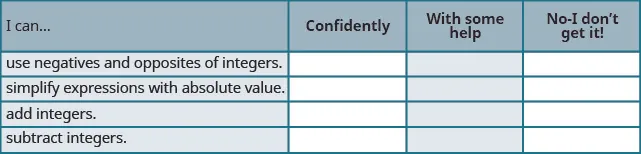What does this checklist tell you about your mastery of this section? What steps will you take to improve?

Order a print copy

As an Amazon Associate we earn from qualifying purchases.